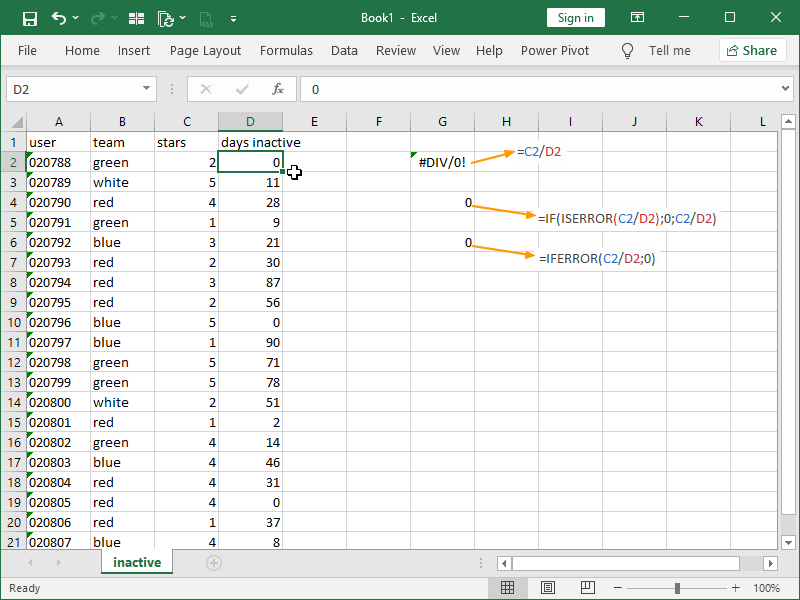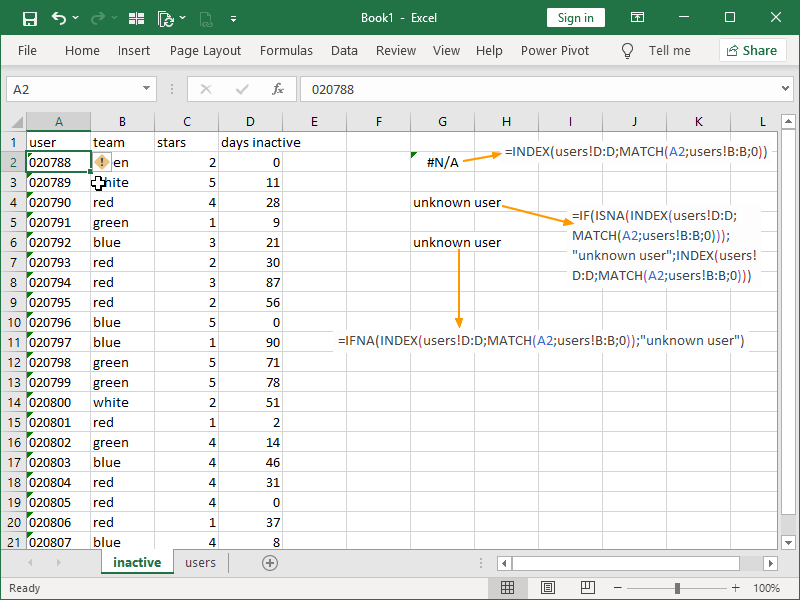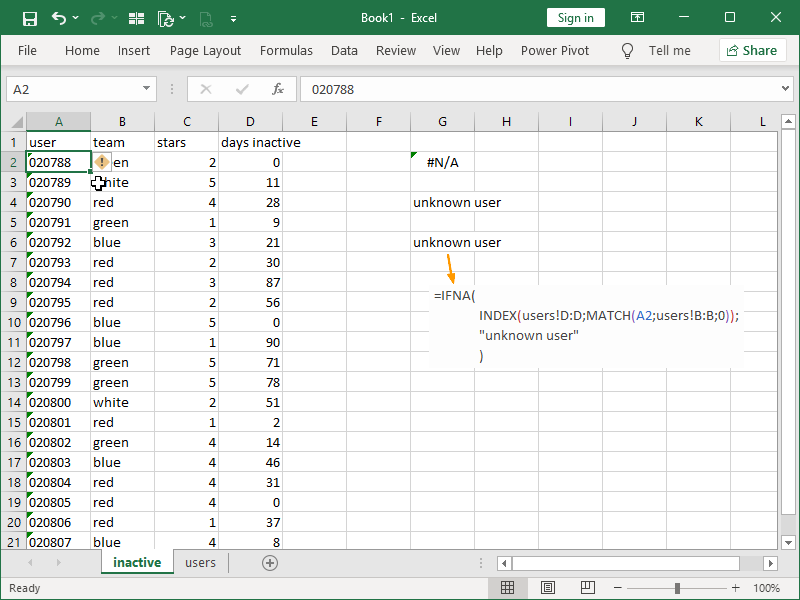# IFERROR and IFNA functions

While effective, this can make the whole formula longer than necessary and hard to read/understand/correct.

The specialized IFERROR and IFNA functions with simpler syntax can take the place of the IF ISERROR and IF ISNA combinations.

The IFERROR function checks whether the cell or formula return is an error (#N/A, #VALUE!, #REF!, #DIV/0!, #NUM!, #NAME?, or #NULL!), and depending on the outcome:

• if there is no error, returns the value from a cell checked or the result of the formula checked,
• if there is an error, returns the value specified.

The syntax of the IFERROR function is as follows:

= IFERROR ( value; value_if_error )

With the IFERROR function, we only have to enter the formula we are checking for errors once:The IFNA function checks whether the cell or formula return is an #N/A error, and depending on the outcome:

• if there is no #N/A error, returns the value from a cell checked or the result of the formula checked,
• if there is an #N/A error, returns the value specified.

The syntax of the IFNA function is as follows:

= IFNA ( value; value_if_na )

Specifically, the IFNA function is often used to test the returns of the INDEX MATCH combination, given that the #N/A error will be retuned when a match cannot be found:If readability is the goal, we can also add line breaks to the IFNA function arguments:Dig deeper:

Conditional statements